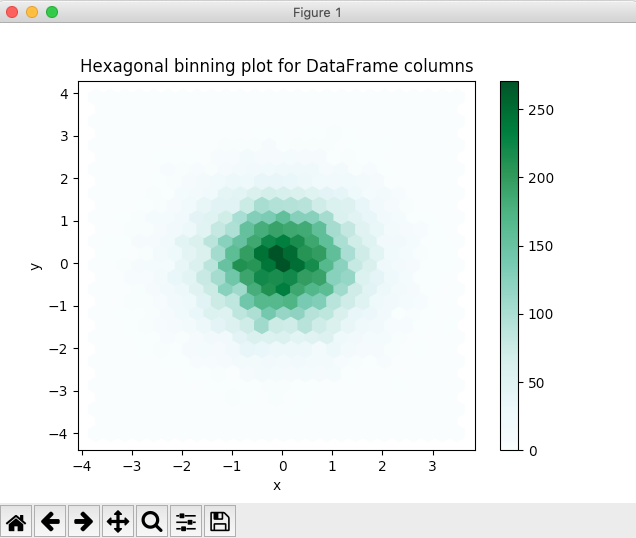# Drawing A Hexagonal Binning Plot Using Pandas DataFrame

## Overview:

• A two-dimensional lattice is divided into a number of hexagonal grids of size (nxm).
• Based on the number of data points of the distributions of two variables x,y that fall under any hexagon, the hexagons are given a color ramp or given different sizes.
• A hexagonal binning plot is drawn when the number of points in the distribution is large.

## Plotting a Hexagonal Binning Plot for a Pandas DataFrame:

• The method hexbin() of the plot member of a DataFrame instance draws a hexagonal binning plot for set of (x,y) points.

## Example:

 # Example python program to draw a Hexagonal Binning chart # for a pandas DataFrame import numpy as np import pandas as pd import matplotlib.pyplot as plot   # Generate two distributions of very large size distribution2d  = np.random.randn(20000, 2); dataFrame       = pd.DataFrame(data=distribution2d, columns=["x", "y"]);   # Draw a hexagonal binning chart dataFrame.plot.hexbin(x='x', y='y', gridsize=25, title="Hexagonal binning plot for DataFrame columns"); plot.show(block=True);

## Output: